## Tuesday, March 3, 2015

### The trade-off between mean income and Gini in a welfarist framework

1. The question
Over the last century and a half, global inequality has increased from approximately a Gini of 55 to a Gini of 70. Assuming that (1) incomes in the world are distributed lognormally, and (2) that individual welfare is a logarithmic function of income, we ask the following question: is the increase in mean global income which has occurred over the same period  sufficient to have compensated for the increase in inequality, measured by the Gini?
2. Welfare, mean income, and dispersion of a distribution
2a. Welfare, mean income and standard deviation of logs of income
Incomes (y) are a (non-transformed) variable that follows a lognormal distribution with the mean μ and the standard deviation σ,  that is y:Λ(μ,σ).
Consequently, the transformed variable log y is normally distributed with the mean m and standard deviation s, that is log y: N(m,s).
Note that m and s will be small values because they are the logs of a variable like income. Thus, the mean income μ will be a much larger number than m which is the mean of logs of income.  The same is true for σ,  the standard deviations  of actual incomes, which is a large number as opposed to s which is a small number-- a standard deviation of logs of income.
The  following two relationships hold:
The mean of the lognormal distribution, i.e. the mean of actual incomes, is: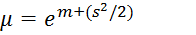(1)
And variance of actual incomes,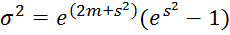Thus,  ln μ = m+(s2/2)  and so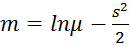(2)
The last is a standard relationship.  It is crucial for our purposes because m is the mean (expected value) of logs of income. Thus, if utility is a logarithmic function of income (W=log y), then m will be the expected value of utility (i.e., mean welfare in a community);  ln μ is simply the logarithm of mean income and s is standard deviation  of logs of income.  (Notice again that s will be a very small number.)
2b. Compensatory increase in income derived
Now, we can easily calculate the indifference condition, namely a condition such that is the increase in mean income is just sufficient (from the welfare point of view) to offset an increased dispersion of incomes, measured by s.
Thus, from (2),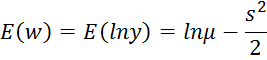and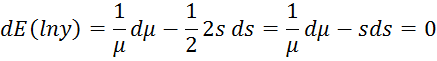and the condition becomes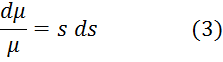We call this compensatory income change. It is the percentage increase in mean income needed to offset (compensate), from the welfare point of view, a given increase in the dispersion of (logs) of incomes. (Obviously, the reverse holds too: if s goes down, compensatory income change will be negative.)
As equation (3) shows, the compensatory income change is increasing in s. In other words, if there is a given change in s (ds=given), the compensatory increase in mean income is the greater, the greater the starting position s, that is the initial dispersion of incomes. Thus, at higher levels of inequality—measured by s—compensatory increases in mean income have to be progressively greater.
3.Gini and standard deviation of lognormal income distribution
Now, independently, we know that when incomes are lognormally distributed,  Gini is related to the standard deviation of incomes as follows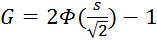(4)

where Φ is a cumulative distribution function of a normally distributed variable with the mean = 0 and standard deviation = 1. For example if Gini = 0.5, then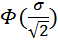=0.75 which is the case if s=0.96. (Note again that s is the standard deviation of logs of income.)
If we could write out s explicitly  as a function of Gini, we could directly substitute s by Gini in equation (3) and thus directly link compensatory income change to Gini. This is our objective but we shall have to reach it in a roundabout way because it is hard (impossible?) to write out s as an explicit function of Gini.
We have to tease out numerically the nature of the relationship between  s and Gini.  Figure 1 shows the relationship: we let Gini increase from 0 to 1 and find the corresponding  s’s  from equation (4). It is obvious from the Figure that Gini is concave in s, that is that ever greater increases in s are needed to elicit a given increase in Gini—or differently put, a given increase in Gini (at high Gini values) implies ever greater increases in s.

Figure 1. The numerical  relationship between standard deviation of log of incomes and Gini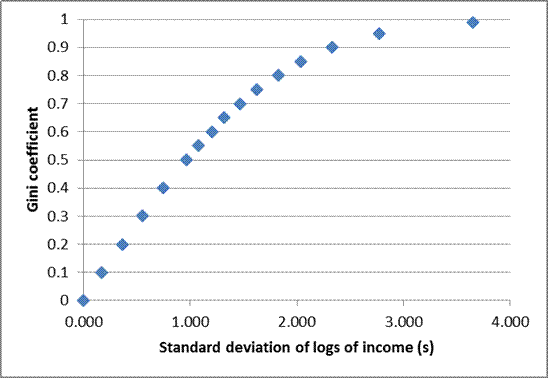This means that the trade-off between mean income and Gini is even sharper than the trade-off  between mean income and standard deviation of logs (s) as given by (3).  As shown in Figure 1, up to approximately G of 0.5, the changes in s and G are about equi-proportionate, and we can write approximately ds=K0 dG where K=some constant. Thus, up to  G≈0.5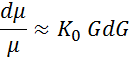Note  that this still implies that compensatory income  change is increasing in initial inequality (initial G).  But at values of Gini greater than 0.5, the compensatory income changes  are even greater than those implied by this last relationship because of the concavity of Gini with respect to s.  At such high values of Gini, a given percentage increase of s will move Gini by very little. Gini will be very sluggish. Thus, at these  high Gini values, a given change in Gini will have to be compensated by even greater increases in mean income.
In other words, at very  high levels of inequality, we need huge (and ultimately infinite) increases in mean income to offset further increases in Gini. This should be  intuitively obvious:  as Gini approaches 1, it will requires enormous increases in mean income to keep the overall welfare unchanged simply because more and more of total income will accrue to fewer and fewer people. The welfare gains from income gains are minimal while welfare losses for those who lose out are much larger. To balance off the two (gains and losses), we need a very large increase in overall, and thus average, income.
4. What is compensatory income change for a long-term increase in global inequality?
We can now answer the original question: if global Gini had increased from about 0.55 around 1870 to 0.7 today,  what is the compensatory increase in average world income?  The standard deviations of log of incomes corresponding to the Ginis of 0.55 and 0.7 are respectively 1.075 and 1.471 (from equation 4). If we use  equation (3)—which is indeed derived for infinitesimal changes, but will be used here in the case of a discrete change—we get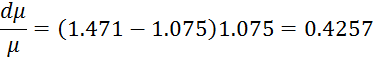which means that the compensatory mean income increase is 42.57 percent. Around 1870, the mean income (GDP per capita) of the world was some \$PPP 911. The compensatory increase would have been about \$PPP 388, that is the mean global income would have to rise to about \$PPP 1,300 for the overall average welfare to remain unchanged.  However, today’s GDP per capita is \$PPP 6,200 or almost five times greater than the compensatory income level would have to be.  So, within this framework, it is obvious that the average world welfare must be much greater today than in 1870.
Incidentally. it also shows why at Gini levels in excess of about 0.5, compensatory income increases have to be (at times perhaps) inordinately high in order to keep aggregate welfare unchanged.
5. How does it look in terms of Sen’s index?
Sen’s welfare index, interpreted as total welfare of a community, is defined as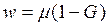It thus provides a direct relationship between mean income and Gini.  Using total differention of dw as before to find the compensatory income change we obtain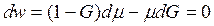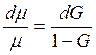The last relationship provides a direct link between the change in Gini and compensatory change in mean income. Are the results similar to what we have obtained before?  We readily see that at high values of Gini, compensatory income increases (with a given dG) become greater. Similarly, when G approaches 1, the compensatory income increases tends (like in our case) toward very high values, even infinity. Using the same global data as before, we find that the compensatory income increase which would have kept  Sen’s  welfare index unchanged, is 0.33 or 33 percent.  Thus with Sen’s index we get that the result that, to offset global increase in inequality from 0.55 to 0.7, mean  global income had to go up by 33 percent, that is less than some 43 percent that we found earlier. Obviously, in both cases, the overall mean welfare today must be significantly greater than in 1870.

 We normalize throughout by total population, i.e., do not take into account population increase which may be valuable, in total welfare sense, by itself (see Jean-Yves Duclos).